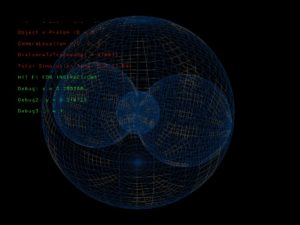# Research

Below are areas of research I’m currently engaged in with useful notes for my self and others collaborating with me

CFD(Computational Fluid Dynamics)

I’m trying to build a virtual wind tunnel to work out the Drag Coefficient of 3D shapes from mach 0-30.

Electrostatics

https://en.wikipedia.org/wiki/Interatomic_potential

https://en.wikipedia.org/wiki/Morse_potential

https://en.wikipedia.org/wiki/Lennard-Jones_potential

https://en.wikipedia.org/wiki/Molecular_dynamics

https://en.wikipedia.org/wiki/Molecular_mechanics

https://en.wikipedia.org/wiki/Electric_potential

https://en.wikipedia.org/wiki/Electrostatics

https://en.wikipedia.org/wiki/Multipolar_exchange_interaction

The above link would be useful for simulating magnetic field interactions between particles

CFD

https://en.wikipedia.org/wiki/Computational_fluid_dynamics

https://en.wikipedia.org/wiki/Navier%E2%80%93Stokes_equations

https://en.wikipedia.org/wiki/Euler_equations_(fluid_dynamics)

Reynolds Number

Thermodynamics

I’m trying to simulate the flow of heat for objects flying through a fluid at high speeds.

Atomic Orbital Scale and Shape

I’m trying to visualize the shapes of atoms in realistic 3D but the size of the various electron shape types S,P,D etc has only been solved for hydrogen. I need a formula to guesstimate the sizes of electron shapes for non hydrogen atoms. Even if it’s only a few elements from the periodic table ie what is the radius of 1S for Helium? This shell should contain 2 electrons, how many fempto or pico meters in radius is it from the center of the helium atom?

Below: Rendering of an atom using C++ OpenGL